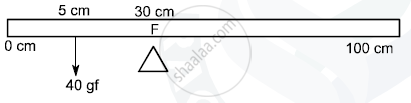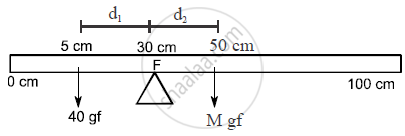# (I) Calculate the Weight of the Meter Scale. (Ii) Which of the Following Options is Correct to Keep the Ruler in Equilibrium When 40 Gf Wt is Shifted to 0 Cm Mark ? F is Shifted Towards 0 Cm - Physics

Numerical

A uniform meter scale is in equilibrium as shown in the diagram :(i) Calculate the weight of the meter scale.
(ii) Which of the following options is correct to keep the ruler in equilibrium when 40 gf wt is shifted to 0 cm mark ?
F is shifted towards 0 cm
or
F is shifted towards 100 cm

#### Solution(i) Mass of the meter scale wiil be concentrated at its centre
∴ At 50 cm, Mass of meter scale = M gf
therefore F_1d_1= F_2 d_2
(25) = F_2(20)

50 gf = F2

0.49N = F

∴ F2 = m (9.8)

=(0.49)/(9.8)= m

∴ m = 0.05kg

1m = 500m

(ii) F is shifted towards 0 cm.

Concept: Equilibrium of Bodies and Its Types
Is there an error in this question or solution?